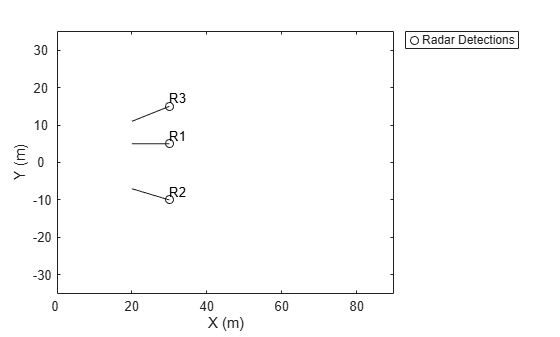Main Content

# plotDetection

Plot set of detections in theater detection plotter

## Syntax

``plotDetection(detPlotter,positions)``
``plotDetection(detPlotter,positions,velocities)``
``plotDetection(detPlotter,positions,___,labels)``
``plotDetection(detPlotter,positions,___,covariances)``

## Description

````plotDetection(detPlotter,positions)` specifies positions of M detected objects whose positions are plotted by the detection plotter `detPlotter`. Specify the positions as an M-by-3 matrix, where each column of the matrix corresponds to the x-, y-, and z-coordinates of the detected object locations.```
````plotDetection(detPlotter,positions,velocities)` also specifies the corresponding velocities of the detections. Velocities are plotted as line vectors emanating from the center positions of the detections. If specified, `velocities` must have the same dimensions as `positions`.```

example

````plotDetection(detPlotter,positions,___,labels)` also specifies a cell vector of length M whose elements contain the text labels corresponding to the M detections specified in the positions matrix. If omitted, no labels are plotted.```
````plotDetection(detPlotter,positions,___,covariances)` also specifies the covariances of the M detection uncertainties, where the covariances are a 3-by-3-by-M matrix of covariances that are centered at the positions of each detection. The uncertainties are plotted as an ellipsoid```

## Examples

collapse all

Create a theater plot.

`tp = theaterPlot('XLim',[0,90],'YLim',[-35,35],'ZLim',[1,10]);`

Create a detection plotter with the name `Radar Detections`.

`radarPlotter = detectionPlotter(tp,'DisplayName','Radar Detections');`

Update the detection plotter with three detections labeled `'R1'`, `'R2'`, and `'R3'` positioned in units of meters at $\left(30,5,4\right)$, $\left(30,-10,2\right)$, and $\left(30,15,1\right)$ with corresponding velocities (in m/s) of $\left(-10,\text{\hspace{0.17em}}0,\text{\hspace{0.17em}}2\right)$, $\left(-10,\text{\hspace{0.17em}}3,\text{\hspace{0.17em}}1\right)$, and $\left(-10,\text{\hspace{0.17em}}-4,1\right)$, respectively.

```positions = [30, 5, 4; 30, -10, 2; 30, 15, 1]; velocities = [-10, 0, 2; -10, 3, 1; -10, -4, 1]; labels = {'R1','R2','R3'}; plotDetection(radarPlotter, positions, velocities, labels)```## Input Arguments

collapse all

Detection plotter, specified as a `detectionPlotter` object.

Detection positions, specified as an M-by-3 real-valued matrix, where M is the number of detections. Each column of the matrix corresponds to the x-, y-, and z-coordinates of the detection positions in meters.

Detection velocities, specified as an M-by-3 real-valued matrix, where M is the number of detections. Each column of the matrix corresponds to the x-, y-, and z-velocities of the detections. If specified, `velocities` must have the same dimensions as `positions`.

Detection labels, specified as a M-by-1 cell array of character vectors, where M is the number of detections. The input argument `labels` contains the text labels corresponding to the M detections specified in `positions`. If `labels` is omitted, no labels are plotted.

Detection uncertainties of M tracked objects, specified as a 3-by-3-by-M real-valued array of covariances. The covariances are centered at the positions of each detection and are plotted as an ellipsoid.

## See Also

Introduced in R2018b

## Support

#### Sensor Fusion and Tracking for Autonomous Systems

Download white paper